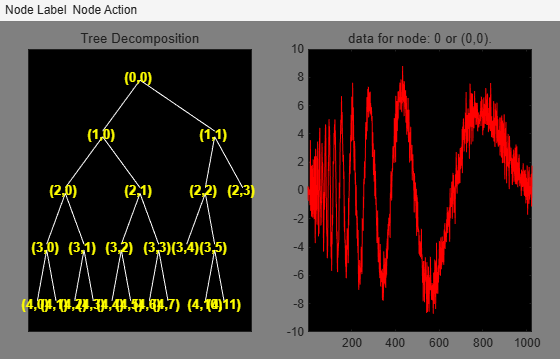# besttree

Best tree wavelet packet analysis

## Syntax

``B = besttree(T)``
``[B,E] = besttree(T)``
``[B,E,N] = besttree(T)``

## Description

`besttree` is a one- or two-dimensional wavelet packet analysis function that computes the optimal subtree of an initial tree with respect to an entropy type criterion. The resulting tree may be much smaller than the initial one.

example

````B = besttree(T)` returns the best tree `B` of the wavelet packet tree `T` corresponding to the best entropy value.```
````[B,E] = besttree(T)` also returns the best entropy value `E`.```
````[B,E,N] = besttree(T)` also returns the indices `N` of the merged nodes.```

## Examples

collapse all

This example shows to obtain the optimal wavelet packet tree based on an entropy criterion.

Load the noisy Doppler signal. Save the current extension mode, and then change to the periodic extension mode. Obtain the wavelet packet tree down to level 4 with the `'sym4'` wavelet.

```load noisdopp origMode = dwtmode('status','nodisp'); dwtmode('per','nodisp') T = wpdec(noisdopp,4,'sym4');```

Obtain the best wavelet packet tree and plot the result.

```BstTree = besttree(T); plot(BstTree)```Restore the DWT extension mode to the original setting.

`dwtmode(origMode,'nodisp')`

## Input Arguments

collapse all

Wavelet packet tree, specified as a `wptree` object.

## Output Arguments

collapse all

Best tree, returned as a `wptree` object. `B` may be much smaller than `T`.

Following the organization of the wavelet packets library, it is natural to count the decompositions issued from a given orthogonal wavelet.

A signal of length N = 2L can be expanded in α different ways, where α is the number of binary subtrees of a complete binary tree of depth L. As a result, we can conclude that α ≥ 2N/2 (for more information, see ). This number may be very large, and since explicit enumeration is generally intractable, it is interesting to find an optimal decomposition with respect to a convenient criterion, computable by an efficient algorithm. We are looking for a minimum of the criterion. For more information, see Algorithms.

Optimal entropy of the node, returned as a vector. The optimal entropy of the node, whose index is `j-1`, is `E(j)`.

Merged nodes indices, returned as a vector. `N` contains the indices of the merged nodes.

## Algorithms

Consider the one-dimensional case. Starting with the root node, the best tree is calculated using the following scheme. A node N is split into two nodes N1 and N2 if and only if the sum of the entropy of N1 and N2 is lower than the entropy of N. This is a local criterion based only on the information available at the node N.

Several entropy type criteria can be used (see `wenergy` for more information). If the entropy function is an additive function along the wavelet packet coefficients, this algorithm leads to the best tree.

Starting from an initial tree T and using the merging side of this algorithm, we obtain the best tree among all the binary subtrees of T.

 Coifman, R.R., and M.V. Wickerhauser. “Entropy-Based Algorithms for Best Basis Selection.” IEEE Transactions on Information Theory 38, no. 2 (March 1992): 713–18. https://doi.org/10.1109/18.119732.

 Mallat, Stéphane. “A Wavelet Tour of Signal Processing The Sparse Way.” Elsevier Science & Technology Books, 2009.